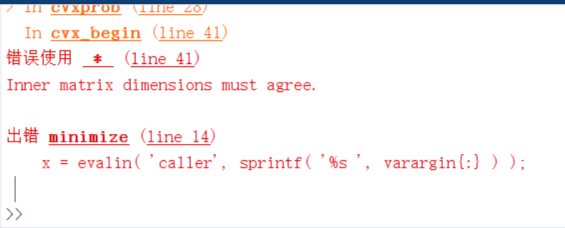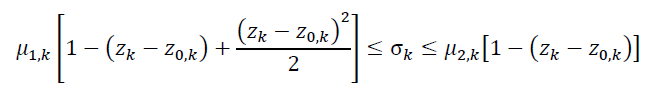# Why a simple problem is failed? Error: Inner matrix dimensions must agree

I am doing a test on a simple convex problem, but the CVX told me “Inner matrix dimensions must agree.”

The code is listed as follows, where x_test is a known 1000 * 1 vector.

cvx_begin
variable z(1000,1) complex
minimize z’*z-real(z’ * x_test)
subject to
norm(z,inf)<=1
cvx_endI have modified the code in the ‘minimize’ line as:

minimize real(z’*z) - real(z’*x_test)

and the code ran successfully.

But I’m wondering if the result is correct?

Use parentheses around the entirety of the objective function. Due to a quirk in CVX or MATLAB (I guess it could be called a feature), it is good practice to always do that when the objective has more than one term, although might not always be necessary.

The following executes successfully.

``````x_test = rand(1000,1);;
cvx_begin
variable z(1000,1) complex
minimize (z'*z-real(z' * x_test))
subject to
norm(z,inf)<=1
cvx_end
``````

If `minimize z'*z-real(z' * x_test)` is used instead, the error message is generated.

``````Error using  *  (line 41)
Inner matrix dimensions must agree.
Error in minimize (line 14)
x = evalin( 'caller', sprintf( '%s ', varargin{:} ) );``````

Thanks a lot!
My problem has been solved by your method.

Hi! I am running into a similar issue, and although I’ve tried the solutions already provided here, it seems that I still get the “Inner matrix dimensions must agree”

What I am trying to implement is the following constraint:where miu1,k, miu2,k and z0,k are scalar inputs, while zk and sigm are one of the variables that I declared.

Additionally, I just allocated the substraction between the z terms in paranthesis as del_z = zk-z0k;
I wrote the constraint in the picture like this:

`miu1k*(1-((del_z)+(((del_z)*(((del_z))/2))))) <= sigm <= miu2k*(1-(del_z));`

I double-checked the dimensions of everything and they are the same:
del_z is 1x101 cvx
sigm is 1x101 cvx

The last term on the LHS is 1 by 101 by 1 by 101, which is non-conformal, hence the error message. Perhaps you want `del_z.^2/2` , which also could be written `del_z.*del_z/2` .

Your use of many extraneous parentheses makes your code unnecessarily difficult to read. Although not responsible for the error message you encountered, it appears that the sign of the last term on the LHS is wrong, because it is insides parentheses preceded by a negative sign.

Thanks for the answer, works nowGood to hear.

Also, you are hereby awarded a free virtual beer for breaking the previous CVX forum record for greatest number of extraneous parentheses.